# Applied Mechanics and Design - Online Test

Q1. The threaded bolts A and B of same material and length are subjected to identical tensile load. If the elastic strain energy stored in bolt A is 4 times that of the bolt B and the mean diameter of bolt A is 12mm, the mean diameter of bolt B in mm is
Explaination / Solution: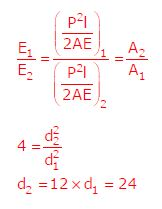Q2. In a rolling process, the state of stress of the material undergoing deformation is
Explaination / Solution:
No Explaination.

Q3. A metric thread of pitch 2mm and thread angle 60º is inspected for its pitchdiameter using 3-wire method. The diameter of the best size wire in mm is
Explaination / Solution:

For 60º thread angle, best wire size =0.57135xP=1.154

Q4. A steel bar 200 mm in diameter is turned at a feed of 0.25 mm/rev with a depth of cut of 4 mm. The rotational speed of the workpiece is 160 rpm. The material removal rate in mm3 / s is
Explaination / Solution:

f × d × vQ5. Which one of following is NOT correct?
Explaination / Solution:
No Explaination.

Q6. Gear 2 rotates at 1200 rpm in counter clockwise direction and engages with Gear 3. Gear 3 and Gear 4 are mounted on the same shaft. Gear 5 engages with Gear 4. The numbers of teeth on Gears 2, 3, 4 and 5 are 20, 40, 15 and 30, respectively. The angular speed of Gear 5 is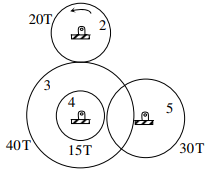Explaination / Solution: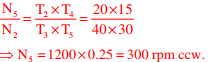Q7. The stress-strain curve for mild steel is shown in the figure given below. Choose the correct option referring to both figure and table.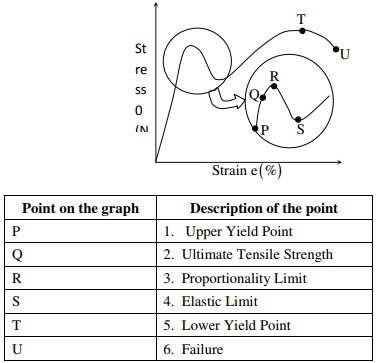Explaination / Solution:
No Explaination.

Q8. A machine produces 0, 1 or 2 defective pieces in a day with associated probability of 1/6, 2/3 and 1/6, respectively. The mean value and the variance of the number of defective pieces produced by the machine in a day, respectively, are
Explaination / Solution:

Let ‘x’ be no. of defective pieces.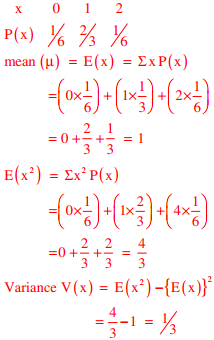Q9. Consider a simply supported beam of length, 50h, with a rectangular cross-section of depth, h, and width, 2h. The beam carries a vertical point load, P, at its mid-point. The ratio of the maximum shear stress to the maximum bending stress in the beam is
Explaination / Solution:

Given, b = 2h, d = h, l = 50h, force = p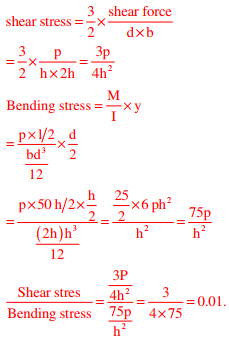Q10. An annular disc has a mass m, inner radius R and outer radius 2R. The disc rolls on a flat surface without slipping. If the velocity of the centre of mass is v, the kinetic energy of the disc is
Explaination / Solution:
No Explaination.